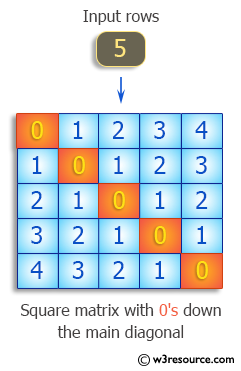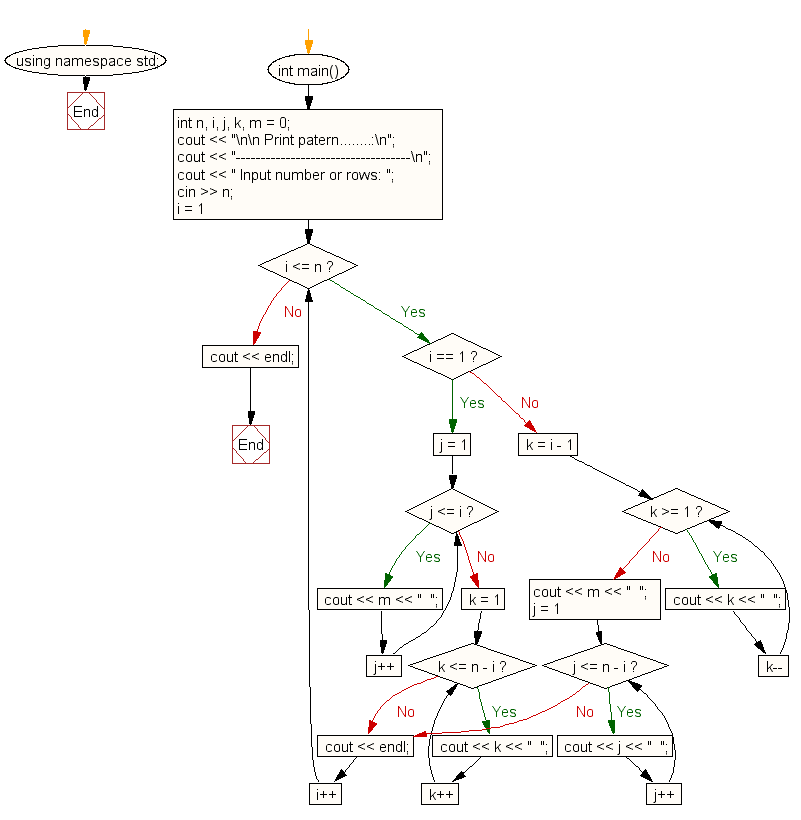﻿ C++ : Produce a square matrix with 0s down the main diagonal# C++ Exercises: Produce a square matrix with 0's down the main diagonal

## C++ For Loop: Exercise-69 with Solution

Write a program in C++ to produce a square matrix with 0's down the main diagonal, 1's in the entries just above and below the main diagonal, 2's above and below that, etc.
0 1 2 3 4
1 0 1 2 3
2 1 0 1 2
3 2 1 0 1
4 3 2 1 0

Pictorial Presentation:Sample Solution:-

C++ Code :

``````#include <iostream>
using namespace std;
int main()
{
int n, i, j, k, m = 0;
cout << "\n\n Print patern........:\n";
cout << "-----------------------------------\n";
cout << " Input number or rows: ";
cin >> n;
for (i = 1; i <= n; i++) {
if (i == 1) {
for (j = 1; j <= i; j++) {
cout << m << "  ";
}
for (k = 1; k <= n - i; k++) {
cout << k << "  ";
}
}
else {
for (k = i - 1; k >= 1; k--) {
cout << k << "  ";
}
cout << m << "  ";
for (j = 1; j <= n - i; j++) {
cout << j << "  ";
}
}
cout << endl;
}
cout << endl;
}
``````

Sample Output:

``` Print patern........:
-----------------------------------
Input number or rows: 8
0  1  2  3  4  5  6  7
1  0  1  2  3  4  5  6
2  1  0  1  2  3  4  5
3  2  1  0  1  2  3  4
4  3  2  1  0  1  2  3
5  4  3  2  1  0  1  2
6  5  4  3  2  1  0  1
7  6  5  4  3  2  1  0
```

Flowchart:C++ Code Editor:

Contribute your code and comments through Disqus.

What is the difficulty level of this exercise?

﻿

## C++ Programming: Tips of the Day

What is a smart pointer and when should I use one?

This answer is rather old, and so describes what was 'good' at the time, which was smart pointers provided by the Boost library. Since C++11, the standard library has provided sufficient smart pointers types, and so you should favour the use of std::unique_ptr, std::shared_ptr and std::weak_ptr.

There was also std::auto_ptr. It was very much like a scoped pointer, except that it also had the "special" dangerous ability to be copied - which also unexpectedly transfers ownership.

It was deprecated in C++11 and removed in C++17, so you shouldn't use it.

```std::auto_ptr<MyObject> p1 (new MyObject());
std::auto_ptr<MyObject> p2 = p1; // Copy and transfer ownership.
// p1 gets set to empty!
p2->DoSomething(); // Works.
p1->DoSomething(); // Oh oh. Hopefully raises some NULL pointer exception.
```

Ref : https://bit.ly/3mc9GHE